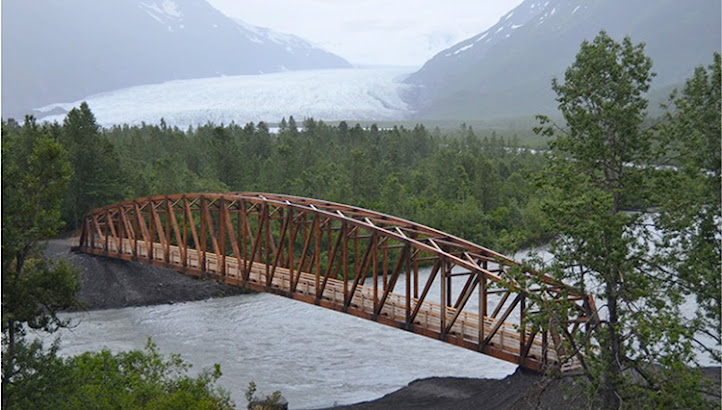Statics

Statics is the basis for the study of engineering mechanics and specifically rigid-body mechanics. Statics is concerned with the equilibrium of bodies that are at rest or that move at a constant velocity. Using measurements of geometry and force, Archimedes studied statics concepts in ancient Greece. Most of his work centered on simple machines for construction of buildings.

In this lesson students will learn how to identify and calculate forces acting on a body when it is in static equilibrium. Students will calculate internal and external forces of a truss. They will use this knowledge to design, build, and test their own truss designs.

K1 – Differentiate between scalar and vector quantities.
K2 – Identify magnitude, direction, and sense of a vector.

K3 – Know beam deflection is related to cross sectional geometry and material properties.

K4 – Know the moment of inertia is related to cross sectional geometry.

K5 – Know the modulus of elasticity defines the stiffness of an object related to material and chemical properties.

K6 – Know the forces acting on a static object are in equilibrium.

K7 – Understand how Newton’s Laws are applied to determine the forces acting on an object.

S1 – Create free body diagrams of objects, identifying all forces acting on the object.
S2 – Mathematically locate the centroid of structural members.
S3 – Calculate the area moment of inertia of structural members.
S4 – Calculate the deflection of a center-loaded beam from the beam’s geometry and material properties.
S5 – Calculate the x- and y-components of a given vector.
S6 – Calculate moments or torques given a force and a point of application relative to a specified axis.
S7 – Use equations of equilibrium to calculate unknown external forces on a truss.
S8 – Use the method of joints to calculate tension and compression forces in the members of a statically determinate truss.
S9 – Construct and destructively test a truss, and relate observations to calculated predictions.

Resources

All About Vectors - website (physicsclassroom.com) - read Lessons 1 & 3 (see the Khan Academy video below for help with Lesson 3)

Force Vectors (and the meaning of a vector's sense) - website (mit.edu)

Free Body Diagrams (FBDs) - website (physicsclassroom.com)

Drawing Free Body Diagrams - YouTube video1 and video2 (Bozeman Science)

Solving for Forces Using a FBD (static case) - YouTube video (Khan Academy)

Torque (aka Moment) - YouTube video (Bozeman Science)

Moment (aka Torque) - website (mit.edu)

Centroid, Force and Torque (notes and practice problems) - worksheets (Google Drive)

Area Moment of Inertia - website (Engineering Toolbox) - don't bother memorizing these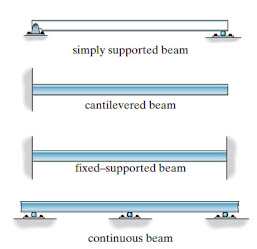Beams are typically straight horizontal members used primarily to carry vertical loads. They may be classified by how they are supported, as we see in the picture at right. (image: Structural Analysis, Hibbeler)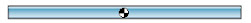The centroid of a beam is its geometric center, indicated on the beam below. For a beam of uniform density, the centroid is also the center of mass.

If a force is applied to the beam such that the line of the force does not pass through the beam's centroid, the beam will rotate about its centroid (and translate, as well). A force through the centroid leads to translation without rotation.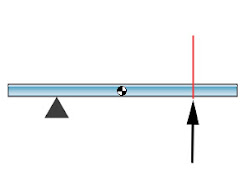If a beam is attached to a pivot or joined to another beam or structure, a force (or torque) will tend to rotate the beam about the pivot or joint (not the centroid). The centroid still serves a purpose, however. In structural analysis (say, using a FBD), the weight of a beam can be represented by a single equivalent force acting at its centroid. Furthermore, the centroid is often needed to calculate the "moment of inertia" for a beam, which you'll read about below. We, however, will tend to ignore the weight of a beam in analyses, and we won't dig deeply enough into "moment of inertia" to find the centroid buried in the mathematics, so you'll have little use for the centroid for now.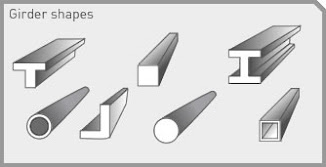This simply supported beam experiences an upward force from each support, a downward force from the boy, and a downward force from its own weight (which we'll ignore). If the beam is to remain stationary, these forces must balance or cancel out.

All beams have the tendency to sag (or deflect). The extent of the deflection is based not only on the load (here, the boy) but also on the material of which the beam is made, along with the beam's moment of inertia (related to its shape).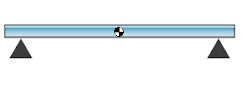Here we see the shape of common beams used in construction. The ability to resist deflection depends partly on this shape via a quantity called moment of inertia. Beware! There are two distinct quantities both often referred to as moment of inertia: one pertains to area, the other to mass. We are concerned with the area moment of inertia (aka the second moment of area).

The larger the area moment of inertia, the less the beam will deflect or sag in the middle. The formula that allows one to calculate deflection based upon moment of inertia (and other variables) will be presented later.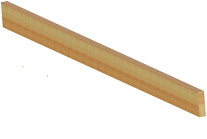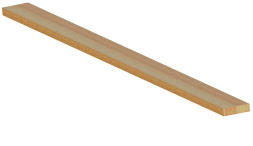(A)

(B)

If we consider the two rectangular beams at left, A and B, and we imagine them supported at their ends, it's easy to visualize beam B deflecting more than beam A under some given load. (Imagine each beam straddling a deep hole. If you stood on A and then B, which would sag more? B, of course.)

Thus, we expect A to have a larger moment of inertia, as the larger the moment of inertia, the less the beam will deflect. The formula for calculating the moment of inertia for a beam of this shape is:

b = the length of the base

h = the length of the height (which is cubed in the                  formula

This peculiar formula is easily derived using calculus, but you won't need to derive it. Notice how beam A, due to its greater "h", ends up with the larger moment.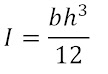Consider again beams A and B, but now imagine that A is made of soft rubber and B is made of steel. Would you still expect A to deflect or sag less?

The composition of a beam affects its deflection under a load. The numerical quantity that captures this vital information is called modulus of elasticity (or Young's modulus). Specifically, it's a measure of how stiff a material is. The stiffer a material, the larger its modulus of elasticity. Thus, we find the same relationship between deflection and modulus of elasticity as we did between deflection and moment of inertia. The larger the elasticity, the less the deflection.The deflection δ of a beam, supported at both ends and with the load at its center (admittedly, a special case), is dependent on:

F = the force or load on the beam

L = the length of the beam

I  = its area moment of inertia

E = its modulus of elasticity

Here's the formula:

Again, calculus is needed to obtain this strange formula, but it should make intuitive sense that F and L are in the numerator and E and I in the denominator.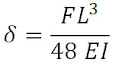The modulus of elasticity is discussed in more detail in Unit 2.3.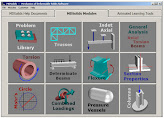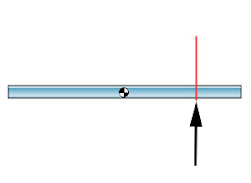Finding the Centroid of an uncommon shape - website (Wikipedia) and tutorial (skyciv.com)

Practice finding the Centroid of various shapes - worksheet (PLTW)

Practice calculating Moment of Inertia and Beam Deflection - worksheet (PLTW)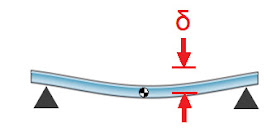The I-beam above (sometimes called an H-beam), has a moment of inertia 3.06 times greater than a solid square beam with equal cross-sectional area. A taller I-beam, with narrower "flanges" at the top and bottom but still the same overall cross-sectional area, has an even greater moment of inertia (and thus a greater resistance to bending). Not surprisingly, I-beams are common in construction.

Let's turn out attention to cantilever beams. They will soon lead us to an understanding of trusses (currently undefined).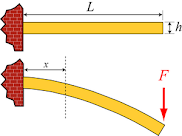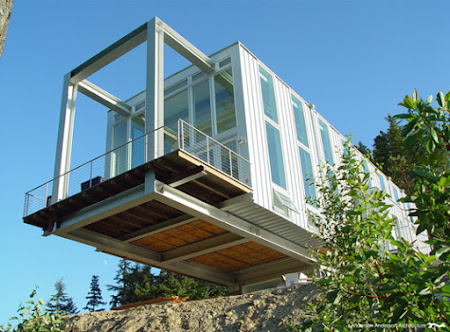Notice the I-beams used in the construction of this cantilever structure.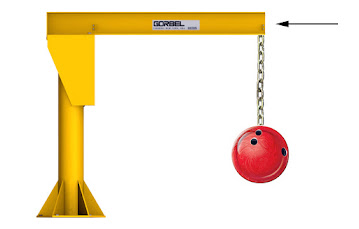The cantilevered beam is supporting the weight of the bowling ball, transferring the load (force), which acts downward, to the vertical support.

The load causes the beam to bend or deflect, which we see exaggerated in the pictures above left as well as below.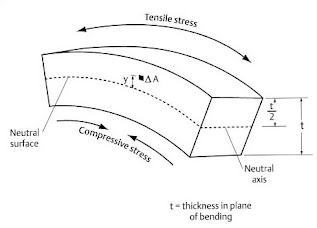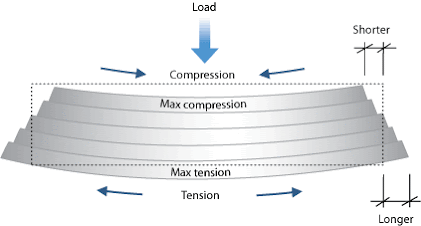The picture at left shows a simply supported beam (not cantilever) with a load applied to its center, for comparison. Notice how it is equivalent to two inverted cantilever beams, side by side.

Be it a simply supported beam, a cantilever, or any other beam supporting a load, a beam will always bend or deflect, causing one of its faces to compress and the other to stretch apart.

For a cantilever, it is the top face that is stretched laterally, its molecules put in tension. We call this lateral stretching a tensile stress, or simply tension.  For a simply supported beam, it is the underside that is placed in tension.

Likewise, for a cantilever, the bottom face is compressed, its molecules forced closer together. This is also the case for the top side of a simply supported beam. We call this a compressive stress or compression.

The middle portion of a deflecting beam is neither stretched nor compressed very much; it therefore can stand to be made thinner than the top and bottom faces. Again, we find a reason for the shape of the popular I-beam.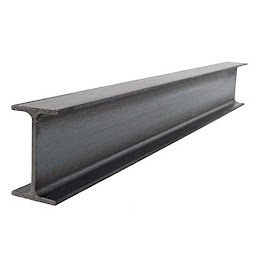Both theory-based analysis and experimentation will reveal that much of the vertical "web" that connects the top and bottom plates or "flanges" is unnecessary. That is, we can remove portions of the web and retain a structurally sound beam. This is effectively what a truss is.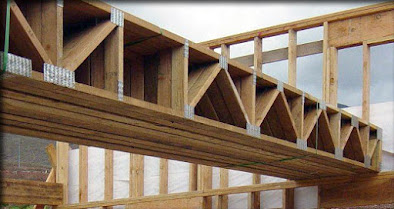A truss is a structure comprised of members, joined together, each in compression or tension, that acts as a single object. It is based upon the geometric rigidity of the triangle, and thus the truss web (akin to the web in an I-beam) is comprised of members oriented either vertically or diagonally (at or near 45 degrees). The "flanges", in the case of a truss, are called chords.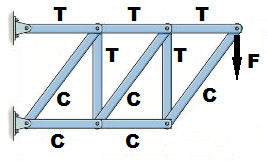Shown at right is a cantilever truss with a load F. Each member of the truss is marked to indicate whether it is in compression (C) or tension (T).

Eventually, you will want to be able to mathematically determine the magnitude of each force, given the load F and the angles between the truss members. In general, this is done using a Free Body Diagram and the understanding that the truss, immobile, has a net force of zero, i.e. all of the forces must cancel out.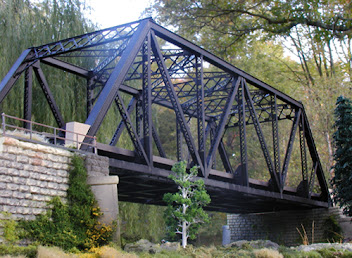Bridges (the medium-sized ones) are often made of trusses, as we see pictured here. There are many different ways one could arrange the vertical and diagonal members. One of the most famous truss designs is called the Pratt truss, patented in 1844 by Thomas and Caleb Pratt. The bridge below is a Pratt truss.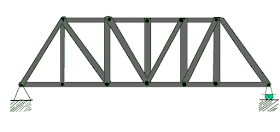Pratt Truss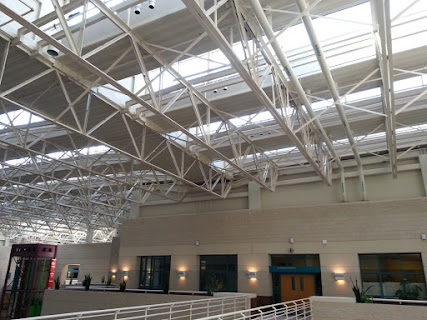Trusses are often used to support ceilings (seen at right), as they can redirect the weight of the ceiling to the walls. Notice that a truss can sit above a loaded surface (as we see with the bridge) or hang below one (as we see with the ceiling).

Trusses, a few facts and designs - website

Practice Problems (Centroids, Finding Forces, Trusses) - worksheet

Build and Test a series of Trusses - handout (PLTW)

Determining Truss ForcesA skyscraper (like the Burj Khalifa in Dubai) is a vertical cantilever! What do you think provides the load on this cantilever?

(The Neutral Axis always passes through the centroid, by the way.)

The maximum deflection of a cantilever, when the load is at the extreme end, is: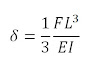Vandegrift High School | 9500 McNeil Drive, Austin, TX | T: 512 570 2300 |  Andrew.Perrone@leanderisd.org